# Electronic Circuits - Negative Clipper Circuits

The Clipper circuit that is intended to attenuate negative portions of the input signal can be termed as a Negative Clipper. Among the negative diode clipper circuits, we have the following types.

• Negative Series Clipper
• Negative Series Clipper with positive $V_{r}$ (reference voltage)
• Negative Series Clipper with negative $V_{r}$
• Negative Shunt Clipper
• Negative Shunt Clipper with positive $V_{r}$
• Negative Shunt Clipper with negative $V_{r}$

Let us discuss each of these types in detail.

## Negative Series Clipper

A Clipper circuit in which the diode is connected in series to the input signal and that attenuates the negative portions of the waveform, is termed as Negative Series Clipper. The following figure represents the circuit diagram for negative series clipper.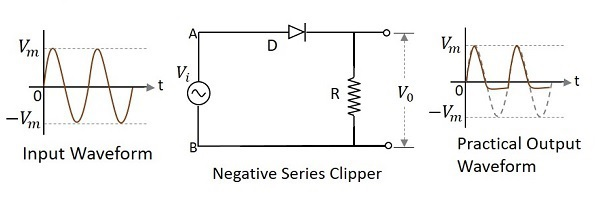Positive Cycle of the Input − When the input voltage is applied, the positive cycle of the input makes the point A in the circuit positive with respect to the point B. This makes the diode forward biased and hence it acts like a closed switch. Thus the input voltage completely appears across the load resistor to produce the output $V_{0}$.

Negative Cycle of the Input − The negative cycle of the input makes the point A in the circuit negative with respect to the point B. This makes the diode reverse biased and hence it acts like an open switch. Thus the voltage across the load resistor will be zero making $V_{0}$ zero.

### Waveforms

In the above figures, if the waveforms are observed, we can understand that only a portion of the negative peak was clipped. This is because of the voltage across $V_{0}$. But the ideal output was not meant to be so. Let us have a look at the following figures.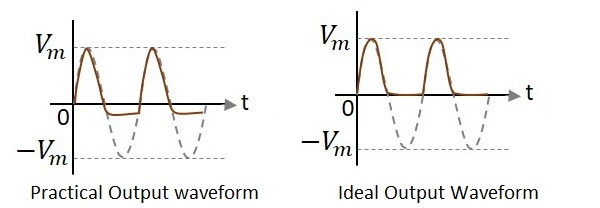Unlike the ideal output, a bit portion of the negative cycle is present in the practical output due to the diode conduction voltage which is 0.7v. Hence there will be a difference in the practical and ideal output waveforms.

### Negative Series Clipper with positive $V_{r}$

A Clipper circuit in which the diode is connected in series to the input signal and biased with positive reference voltage $V_{r}$ and that attenuates the negative portions of the waveform, is termed as Negative Series Clipper with positive $V_{r}$. The following figure represents the circuit diagram for negative series clipper when the reference voltage applied is positive.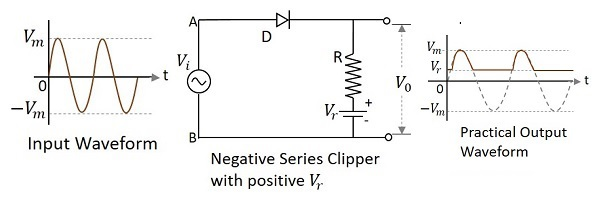During the positive cycle of the input, the diode starts conducting only when the anode voltage value exceeds the cathode voltage value of the diode. As the cathode voltage equals the reference voltage applied, the output will be as shown.

### Negative Series Clipper with negative $V_{r}$

A Clipper circuit in which the diode is connected in series to the input signal and biased with negative reference voltage $V_{r}$ and that attenuates the negative portions of the waveform, is termed as Negative Series Clipper with negative $V_{r}$. The following figure represents the circuit diagram for negative series clipper, when the reference voltage applied is negative.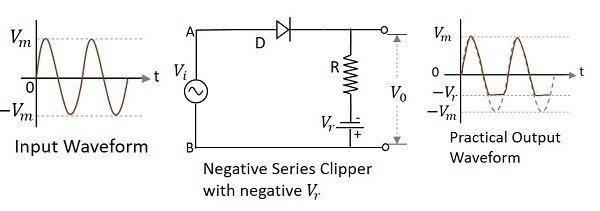During the positive cycle of the input the diode gets forward biased and the input signal appears at the output. During its negative cycle, the diode gets reverse biased and hence will not conduct. But the negative reference voltage being applied, appears at the output. Hence the negative cycle of the output waveform gets clipped after this reference level.

## Negative Shunt Clipper

A Clipper circuit in which the diode is connected in shunt to the input signal and that attenuates the negative portions of the waveform, is termed as Negative Shunt Clipper. The following figure represents the circuit diagram for negative shunt clipper.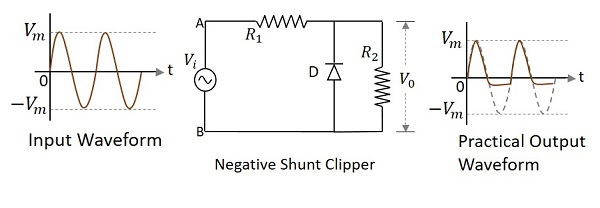Positive Cycle of the Input − When the input voltage is applied, the positive cycle of the input makes the point A in the circuit positive with respect to the point B. This makes the diode reverse biased and hence it behaves like an open switch. Thus the voltage across the load resistor equals the applied input voltage as it completely appears at the output $V_{0}$

Negative Cycle of the Input − The negative cycle of the input makes the point A in the circuit negative with respect to the point B. This makes the diode forward biased and hence it conducts like a closed switch. Thus the voltage across the load resistor becomes zero as no current flows through it.

### Waveforms

In the above figures, if the waveforms are observed, we can understand that just a portion of the negative peak was clipped. This is because of the voltage across $V_{0}$. But the ideal output was not meant to be so. Let us have a look at the following figures.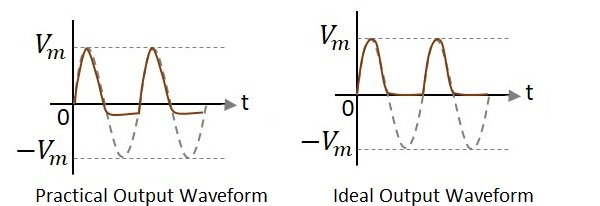Unlike the ideal output, a bit portion of the negative cycle is present in the practical output due to the diode conduction voltage which is 0.7v. Hence there will be a difference in the practical and ideal output waveforms.

### Negative Shunt Clipper with positive $V_{r}$

A Clipper circuit in which the diode is connected in shunt to the input signal and biased with positive reference voltage $V_{r}$ and that attenuates the negative portions of the waveform, is termed as Negative Shunt Clipper with positive $V_{r}$. The following figure represents the circuit diagram for negative shunt clipper when the reference voltage applied is positive.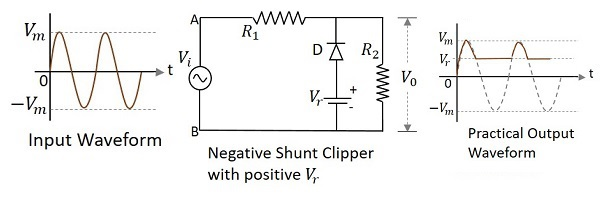During the positive cycle of the input the diode gets reverse biased and behaves as an open switch. So whole of the input voltage, which is greater than the reference voltage applied, appears at the output. The signal below reference voltage level gets clipped off.

During the negative half cycle, as the diode gets forward biased and the loop gets completed, no output is present.

### Negative Shunt Clipper with negative $V_{r}$

A Clipper circuit in which the diode is connected in shunt to the input signal and biased with negative reference voltage $V_{r}$ and that attenuates the negative portions of the waveform, is termed as Negative Shunt Clipper with negative $V_{r}$. The following figure represents the circuit diagram for negative shunt clipper, when the reference voltage applied is negative.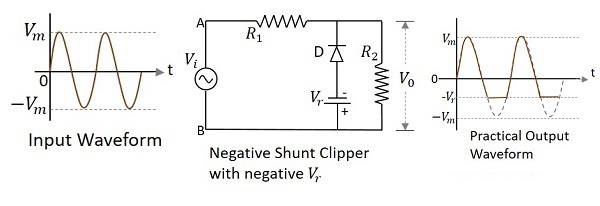During the positive cycle of the input the diode gets reverse biased and behaves as an open switch. So whole of the input voltage, appears at the output $V_{o}$. During the negative half cycle, the diode gets forward biased. The negative voltage up to the reference voltage, gets at the output and the remaining signal gets clipped off.

## Two-way Clipper

This is a positive and negative clipper with a reference voltage $V_{r}$. The input voltage is clipped two-way both positive and negative portions of the input waveform with two reference voltages. For this, two diodes $D_{1}$ and $D_{2}$ along with two reference voltages $V_{r1}$ and $V_{r2}$ are connected in the circuit.

This circuit is also called as a Combinational Clipper circuit. The figure below shows the circuit arrangement for a two-way or a combinational clipper circuit along with its output waveform.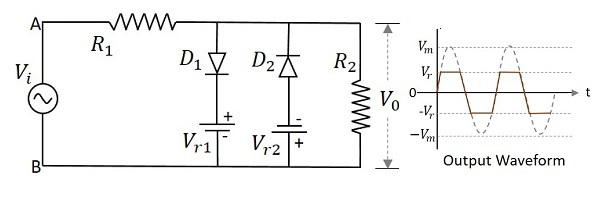During the positive half of the input signal, the diode $D_{1}$ conducts making the reference voltage $V_{r1}$ appear at the output. During the negative half of the input signal, the diode $D_{2}$ conducts making the reference voltage $V_{r1}$ appear at the output. Hence both the diodes conduct alternatively to clip the output during both the cycles. The output is taken across the load resistor.

With this, we are done with the major clipper circuits. Let us go for the clamper circuits in the next chapter.